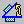# Angle ConstraintsAngle-type constraints fall into three categories.
When defining an angle constraint between planar elements, you need to specify how faces should be oriented.
The offset value is always displayed next to the offset constraint:

• Angle
• Parallelism (when angle value equals zero), when setting a parallelism constraint, green arrows appear on the selected faces to indicate the orientations.
• Perpendicularity (angle value equals 90 degrees)
When setting an angle constraint, you have to define an angle value. Note that this angle value must not exceed 90 degrees.
The tolerance i.e. the smallest angle that can be used to differentiate two elements is set at 10-6 radians.
The following table shows the elements you can select for an offset constraint.Line Plane LinePlane• Line: a line, a cylinder axis, a cone axis for example.
• Plane: a plane or a planar surface for example.